# Class 12 Maths NCERT Solutions for Chapter 8 Application of Integrals Exercise 8.1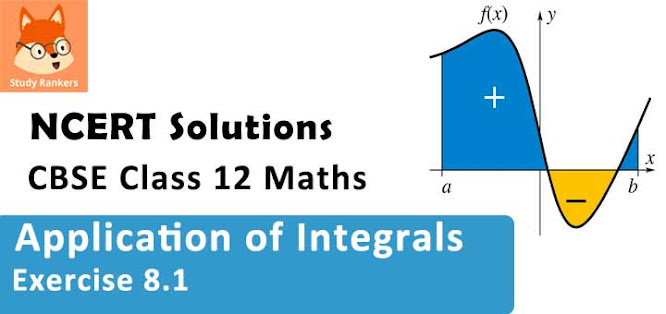### Integrals Exercise 8.1 Solutions

1. Find the area of the region bounded by the curve y2 = x and the lines x = 1, x = 4 and the x - axis.

Solution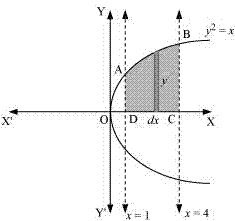The area of the region bounded by the curve, y2 = x, the lines, x = 1 and x= 4, and the x - axis is the area ABCD.
Area of ABCD = ∫14 y dx
=  14 √x dx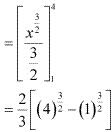= (2/3) [8 - 1]
= 14/3 units

2. Find the area of the region bounded by y2 = 9x, x = 2, x = 4 and the x-axis in the first quadrant.
Solution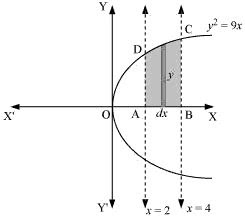The area of the region bounded by the curve, y2 = 9x, x = 2, and x = 4, and the x - axis is the area ABCD.
Area of ABCD = ∫24 y dx
= ∫24 3√x dx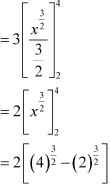= 2[8 - 2√2]
= (16 - 4√2) units

3. Find the area of the region bounded by x2 = 4yy = 2, y = 4 and the y-axis in the first quadrant.
Solution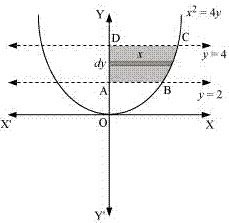The area of the region bounded by the curve, x2 = 4y, y = 2, and y = 4, and the y- axis is the area ABCD.
Area of ABCD = ∫24 y dx
= ∫24 2√y dy
= 2∫24 √y dy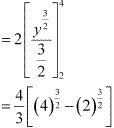= (4/3)[8 - 2√2]
= (32 - 8√2)/3  units

4. Find the area of the region bounded by the ellipse x2/16 + y2/9 = 1
Solution
The given equation of the ellipse, x2 /16 + y2 /9 = 1 , can be represented as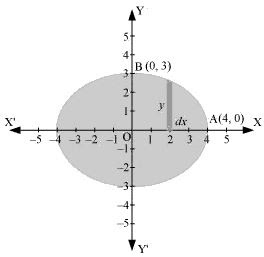It can be observed that the ellipse is symmetrical about x - axis and y - axis.
∴ Area bounded by ellipse = 4× Area of OAB
Area of OAB = ∫24 y dx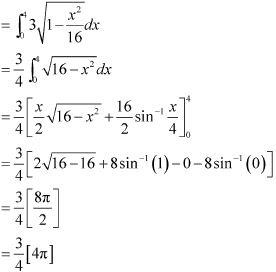Therefore, area bounded by the ellipse = 4 × 3π = 12π units

5. Find the area of the region bounded by the ellipse  x2/4 + y2/9 = 1
Solution
The given equation of the ellipse can be represented as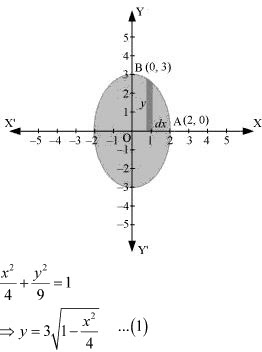It can be observed that the ellipse is symmetrical about x - axis and y - axis.
∴ Area bounded by ellipse = 4 × Area OAB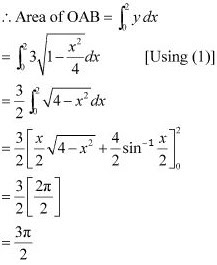Therefore, area bounded by the ellipse = 4× (3π/2) = 6π units

6. Find the area of the region in the first quadrant enclosed by x-axis, line x = √3 y  and the circle x2 + y2 = 4.
Solution
The area of the region bounded by the circle, x2 + y2 = 4, x = √3 y, and the x - axis is the area OAB.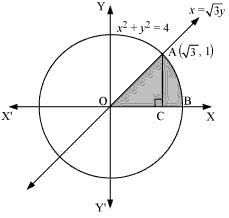The point of intersection of the line and the circle in the first quadrant is (√3, 1) .
Area OAB = Area ΔOCA + Area ACB
Area of OAC = (1/2) × OC × AC = (1/2) × √3 × 1 = √3/2  ...(1)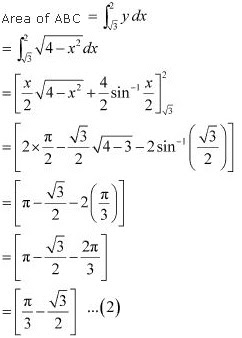Therefore, area enclosed by x - axis, the line x = √3 y, and the circle x2 + y2 = 4 in the
first quadrant = √3/2  + π/2 - √3/2 =  π/3 units

7. Find the area of the smaller part of the circle x2 + y2 = a2 cut off by the line x = a/√2
Solution
The area of the smaller part of the circle, x2 + y2 = a2 , cut off by the line, x = a/√2, is the area ABCD.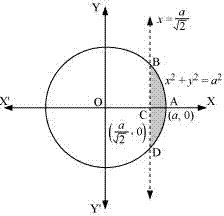It can be observed that the area ABCD is symmetrical about x - axis.
∴ Area ABCD = 2 × Area ABC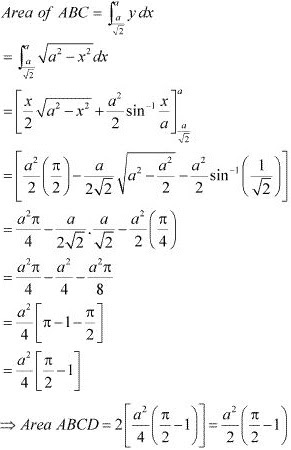Therefore, the area of smaller part of the circle , x2 + y2 = a2 , cut off by the line, x = a/√2, is [(a2 /2)(π/2 - 1)] units.

8. The area between x = y2 and x = 4 is divided into two equal parts by the line x = a, find the value of a.
Solution
The line, x = a, divides the area bounded by the parabola and x = 4 into two equal parts.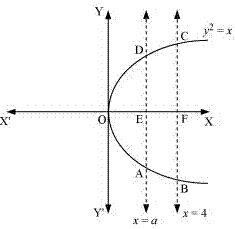It can be observed that the given area is symmetrical about x - axis.
∴ Area OED  = Area EFCD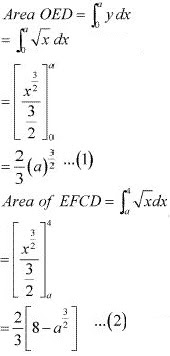From (1) and (2), we obtain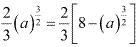⇒ 2.(a)3/2 = 8
⇒ (a)3/2 = 4
⇒ a = (4)2/3
Therefore, the value of a is (4)2/3 .

9. Find the area of the region bounded by the parabola y = x2 and y = |x| .
Solution
The area bounded by the parabola, x2 = y , and the line, y = |x|, can be represented as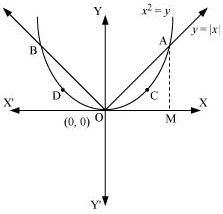The given area is symmetrical about y - axis,
∴ Area OACO = Area ODBO
The point of intersection of parabola, x2 = y, and line, y = x, is A(1, 1).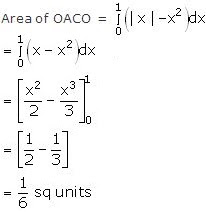Therefore, required area = 2[1/6] = 1/3 sq units

10. Find the area bounded by the curve x2 = 4y and the line x = 4– 2
Solution
The area bounded by the curve, x2 = 4y and line, x = 4y - 2, is represented by the shaded area OBAO.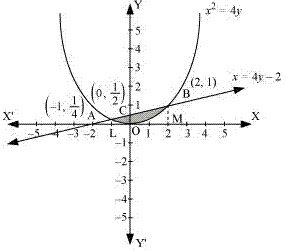Let A and B be the points of intersection of the line and parabola.
Coordinates of point  A are (-1, 1/4).
Coordinates of point B are (2, 1)
We draw AL and BM perpendicular to x - axis.
Similarly, Area OACO = Area OLAC - Area OLAO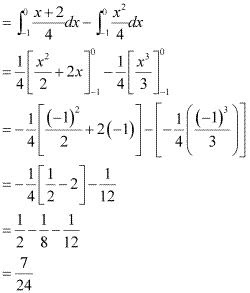Therefore, required area = (5/6 + 7/24) = 9/8 units

11. Find the area of the region bounded by the curve y2 = 4x and the line x = 3
Solution
The region bounded by the parabola, y2 = 4x, and the line, x = 3, is the area OACO.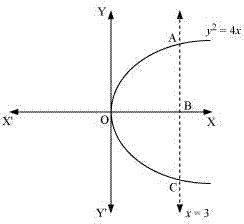The area OACO is symmetrical about x - axis.
Area of OACO = 2 (Area of OAB)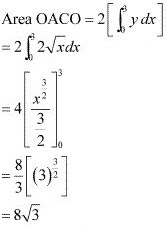Therefore, the required area is 8√3 units.

12. Area lying in the first quadrant and bounded by the circle x2 + y2 = 4 and the lines x = 0 and = 2 is
(A) π
(B) π/2
(C) π/3
(D) π/4
Solution
The area bounded by the circle and the lines, x = 0 and x = 2, in the first quadrant is represented as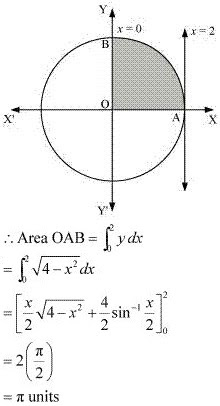Thus, the correct answer is A.

13. Area of the region bounded by the curve y2 = 4x , y - axis and the line y = 3 is
(A) 2
(B) 9/4
(C) 9/3
(D) 9/2
Solution
The area bounded by the curve, y2 = 4x, y - axis, and y = 3  is represented as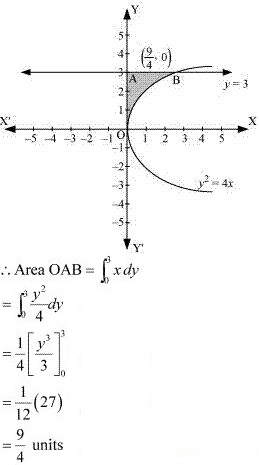Thus, the correct answer is B.